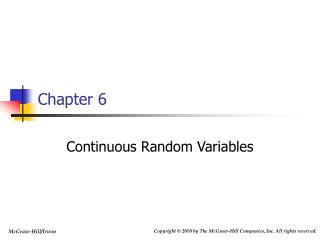Download PresentationChapter 6Chapter 6 - PowerPoint PPT Presentation

Download PresentationChapter 6
An Image/Link below is provided (as is) to download presentation

Download Policy: Content on the Website is provided to you AS IS for your information and personal use and may not be sold / licensed / shared on other websites without getting consent from its author. While downloading, if for some reason you are not able to download a presentation, the publisher may have deleted the file from their server.

- - - - - - - - - - - - - - - - - - - - - - - - - - - E N D - - - - - - - - - - - - - - - - - - - - - - - - - - -
Presentation Transcript

1. Chapter 6 Continuous Random Variables

2. Chapter Outline 6.1 Continuous Probability Distributions 6.2 The Uniform Distribution 6.3 The Normal Probability Distribution 6.4 Approximating the Binomial Distribution by Using the Normal Distribution (Optional) 6.5 The Exponential Distribution (Optional) 6.6 The Normal Probability Plot (Optional)

3. 6.1 Continuous Probability Distributions • A continuous random variable may assume any numerical value in one or more intervals • Use a continuous probability distribution to assign probabilities to intervals of values

4. Continuous Probability Distributions Continued • The curve f(x) is the continuous probability distribution of the continuous random variable x if the probability that x will be in a specified interval of numbers is the area under the curve f(x) corresponding to the interval • Other names for a continuous probability distribution: • Probability curve • Probability density function

5. Properties of Continuous Probability Distributions • Properties of f(x): f(x) is a continuous function such that • f(x) ≥ 0 for all x • The total area under the curve of f(x) is equal to 1 • Essential point: An area under a continuous probability distribution is a probability

6. Area and Probability Figure 6.1

7. 6.2 The Uniform Distribution

8. The Uniform Distribution Continued

9. The Uniform Probability Curve Figure 6.2 (a)

10. 6.3 The Normal Probability Distribution

11. Properties of the Normal Distribution • There are an infinite number of normal curves • The shape of any individual normal curve depends on its specific mean and standard deviation • The highest point is over the mean • Also the median and mode

12. Properties of the Normal Distribution Continued • The curve is symmetrical about its mean • The left and right halves of the curve are mirror images of each other • The tails of the normal extend to infinity in both directions • The tails get closer to the horizontal axis but never touch it

13. Properties of the Normal Distribution Continued • The area under the normal curve to the right of the mean equals the area under the normal to the left of the mean • The area under each half is 0.5

14. Normal Probabilities Figure 6.5

15. Three Important Percentages Figure 6.6

16. Finding Normal Curve Areas

17. Finding Normal Curve Areas Continued Figure 6.7

18. The Cumulative Normal Table Table 6.1

19. Examples Figures 6.8 and 6.9

20. Examples Continued Figures 6.10 and 6.11

21. Examples Continued Figures 6.12 and 6.13

22. Finding Normal Probabilities • Formulate the problem in terms of x values • Calculate the corresponding z values, and restate the problem in terms of these z values • Find the required areas under the standard normal curve by using the table Note: It is always useful to draw a picture showing the required areas before using the normal table

23. Finding a Tolerance Interval Figure 6.23

24. 6.4 Approximating the Binomial Distribution by Using the Normal Distribution(Optional) Figure 6.24

25. Normal Approximation to the Binomial Continued • Suppose x is a binomial random variable • n is the number of trials • Each having a probability of success p • If np 5 and nq 5, then x is approximately normal with a mean of np and a standard deviation of the square root of npq

26. Example 6.8 Figure 6.25

27. 6.5 The Exponential Distribution (Optional) • Suppose that some event occurs as a Poisson process • That is, the number of times an event occurs is a Poisson random variable • Let x be the random variable of the interval between successive occurrences of the event • The interval can be some unit of time or space • Then x is described by the exponential distribution • With parameter λ, which is the mean number of events that can occur per given interval

28. The Exponential Distribution Continued

29. The Exponential Distribution Continued Figure 6.27

30. Example 6.10

31. 6.6 The Normal Probability Plot (Optional) • A graphic used to visually check to see if sample data comes from a normal distribution • A straight line indicates a normal distribution • The more curved the line, the less normal the data is

32. Creating a Normal Probability Plot • Rank order the data from smallest to largest • For each data point, compute the value i/(n+1) • i is the data point’s position on the list • For each data point, compute the standardized normal quantile value (Oi) • Oi is the z value that gives an area i/(n+1) to its left • Plot data points against Oi • Straight line indicates normal distribution

33. Sample Normal Probability Plots Figures 6.30 to 6.32